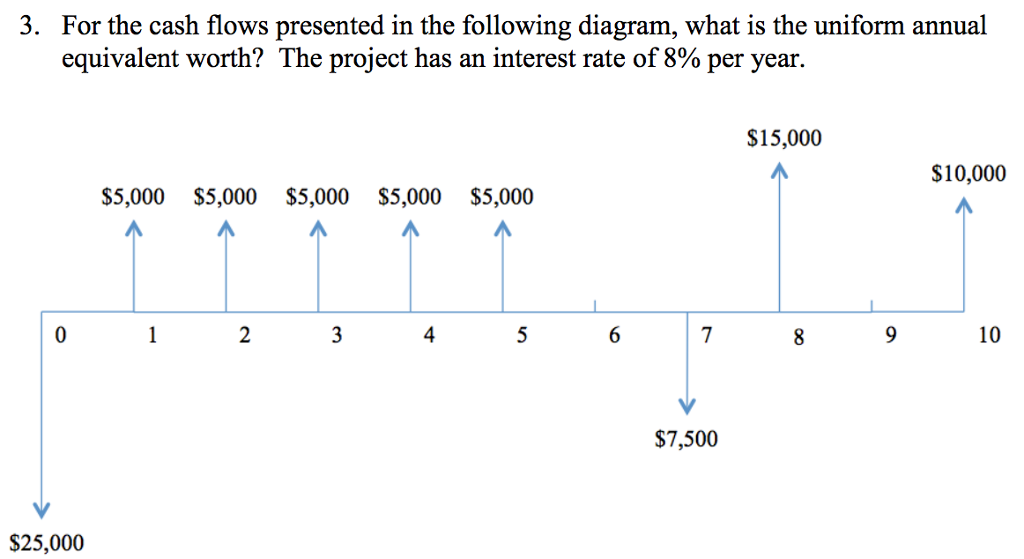# Question & Answer: For the cash flows presented in the following diagram, what is the uniform annual equivalent…..For the cash flows presented in the following diagram, what is the uniform annual equivalent worth? The project has an interest rate of 8% per year.

Present value of cash flows = 5000/1.08 + 5000/1.08^2 + 5000/1.08^3 + 5000/1.08^4 + 5000/1.08^5 + 15000/1.08^8 + 10000/1.08^10 – (25000 + 7500/1.08^7)

Don't use plagiarized sources. Get Your Custom Essay on
Question & Answer: For the cash flows presented in the following diagram, what is the uniform annual equivalent…..
GET AN ESSAY WRITTEN FOR YOU FROM AS LOW AS \$13/PAGE

Present value of cash flows = \$3323.34

Let, equivalent annual worth = EAW

Then,

3323.34 = EAW*(1-1/1.08^10)/.08 = EAW*6.71

EAW = 3323.34/6.71

EAW = \$495.28

Hence, the uniform equivalent annual worth is \$495.28.